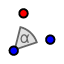# Exploring Rotations

Topic:
Rotation

## Rotate a polygon!

1. Draw a polygon.  Label the vertices by selecting "name" in the text tool.
2. Draw a point on the origin.
3. Rotate your point about the origin using the rotate around a point tool ().  Click on the polygon (shaded area) and then click on the point.  There will be a pop-up that appears asking which direction and what the degree of rotation is.  Rotate the object 3 different times.  First: , then , and  all counter-clockwise.

Which of the following rules would apply to a 90 degree rotation counter-clockwise?

Select all that apply
• A
• B
• C
• D

Which of the following rules would apply to a 180 degree rotation counter-clockwise?

Select all that apply
• A
• B
• C
• D

Which of the following rules would apply to a 270 degree rotation counter-clockwise?

Select all that apply
• A
• B
• C
• D